Main Content

# dsp.FIRRateConverter

Sample rate converter

## Description

The `dsp.FIRRateConverter` System object™ performs sampling rate conversion by a rational factor on a vector or matrix input. The FIR rate convertor cascades an interpolator with a decimator. The rate converter (as shown in the schematic) conceptually consists of an upsampler, followed by a combined anti-imaging and anti-aliasing FIR filter, followed by a downsampler. To design an FIR filter which acts as a combined anti-imaging and anti-aliasing FIR filter, use the `designMultirateFIR` function. For an example, see Resample a Signal using FIR Rate Converter.

The upsampler increases the sample rate of the signal by a factor L and the downsampler reduces the sample rate of the signal by a factor M. Use upsampling and downsampling factors that are relatively prime or coprime. The resulting discrete-time signal has a sample rate that is L/M times the original sample rate.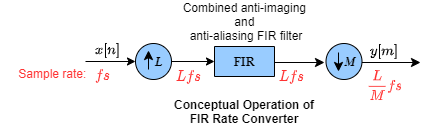Note that the actual object algorithm implements a polyphase structure, an efficient equivalent of the combined system depicted in the diagram. For more details, see Algorithms.

To perform sampling rate conversion:

1. Create the `dsp.FIRRateConverter` object and set its properties.

2. Call the object with arguments, as if it were a function.

To learn more about how System objects work, see What Are System Objects?.

## Creation

### Syntax

``firrc = dsp.FIRRateConverter``
``firrc = dsp.FIRRateConverter(L,M,NUM)``
``firrc = dsp.FIRRateConverter(___,Name,Value)``

### Description

example

````firrc = dsp.FIRRateConverter` returns a FIR sample rate converter, `firrc`, that resamples an input signal at a rate 3/2 times the original sampling rate.```

example

````firrc = dsp.FIRRateConverter(L,M,NUM)` returns an FIR sample rate converter, `firrc`, with the `InterpolationFactor` property set to `L`, the `DecimationFactor` property set to `M`, and the `Numerator` property set to `NUM`.```
````firrc = dsp.FIRRateConverter(___,Name,Value)` returns an FIR sample rate converter, with additional properties specified by one or more `Name,Value` pair arguments.Example: ```firrc = dsp.FIRRateConverter('FullPrecisionOverride','false')``` enables the fixed-point data types to be controlled through the individual fixed-point property settings.```

## Properties

expand all

Unless otherwise indicated, properties are nontunable, which means you cannot change their values after calling the object. Objects lock when you call them, and the `release` function unlocks them.

If a property is tunable, you can change its value at any time.

For more information on changing property values, see System Design in MATLAB Using System Objects.

Interpolation factor L, specified as a positive integer.

Data Types: `single` | `double` | `int8` | `int16` | `int32` | `int64` | `uint8` | `uint16` | `uint32` | `uint64`

Decimation factor M, specified as a positive integer.

Data Types: `single` | `double` | `int8` | `int16` | `int32` | `int64` | `uint8` | `uint16` | `uint32` | `uint64`

Specify the FIR filter coefficients as a row vector in powers of z-1.

The following equation defines the transfer function of the filter:

`$H\left(z\right)=\sum _{n=0}^{N}b\left(n\right){z}^{-n}$`

The vector b = [b(0), b(1), …, b(N)] represents the vector of filter coefficients.

Use a lowpass with normalized cutoff frequency no greater than `min(1/L,1/M)`, where L is the interpolation factor and M is the decimation factor. The gain of the lowpass filter should equal L, the interpolation factor. Choose the length of the filter to be a multiple of the interpolation factor. To design such a filter, use the `designMultirateFIR` function. For an example, see Resample a Signal using FIR Rate Converter. All initial filter states are zero.

Data Types: `single` | `double` | `int8` | `int16` | `int32` | `int64` | `uint8` | `uint16` | `uint32` | `uint64`

### Fixed-Point Properties

Specify whether to use full precision rules. If you set `FullPrecisionOverride` to `true`, which is the default, the object computes all internal arithmetic and output data types using full precision rules. These rules provide the most accurate fixed-point numerics. It also turns off the display of other fixed-point properties because they do not apply individually. These rules guarantee that no quantization occurs within the object. Bits are added, as needed, to ensure that no roundoff or overflow occurs. If you set `FullPrecisionOverride` to `false`, fixed-point data types are controlled through individual fixed-point property settings. For more information, see Full Precision for Fixed-Point System Objects.

Specify the rounding method as one of | `Ceiling` | `Convergent` | `Floor` | `Nearest` | `Round` | `Simplest` | `Zero` |.

#### Dependencies

This property applies only if the object is not in full precision mode.

Specify the overflow action as one of | `Wrap` | `Saturate` |.

#### Dependencies

This property applies only if the object is not in full precision mode.

Specify the filter coefficient fixed-point data type as one of | ```Same word length as input``` | `Custom` |.

Specify the filter coefficient fixed-point type as a `numerictype` (Fixed-Point Designer) object with a `Signedness` of `Auto`.

#### Dependencies

This property applies only when the `CoefficientsDataType` property is `Custom`.

Specify the product fixed-point data type as one of | ```Full precision``` | `Same as input` | `Custom` |.

Specify the product fixed-point type as a scaled `numerictype` (Fixed-Point Designer) object with a `Signedness` of `Auto`.

#### Dependencies

This property applies only when the `ProductDataType` property is `Custom`.

Specify the accumulator fixed-point data type as one of | ```Full precision``` | `Same as product` | ```Same as input``` | `Custom` |.

Specify the accumulator fixed-point type as a scaled `numerictype` (Fixed-Point Designer) object with a `Signedness` of `Auto`.

#### Dependencies

This property applies only when the `AccumulatorDataType` property is `Custom`.

Specify the output fixed-point data type as one of | ```Same as accumulator``` | `Same as product` | ```Same as input``` | `Custom` |.

Specify the output fixed-point type as a scaled `numerictype` (Fixed-Point Designer) object with a `Signedness` of `Auto`.

#### Dependencies

This property applies only when the `OutputDataType` property is `Custom`.

## Usage

### Syntax

``y = firrc(x)``

### Description

example

````y = firrc(x)` resamples the input `x` and returns the resampled signal `y`.```

### Input Arguments

expand all

Data input, specified as a column vector or a matrix. The number of input rows P must be a multiple of the decimation factor M. A P-by-Q matrix input is treated as Q independent channels.

Data Types: `single` | `double` | `int8` | `int16` | `int32` | `int64` | `uint8` | `uint16` | `uint32` | `uint64` | `fi`
Complex Number Support: Yes

### Output Arguments

expand all

Resampled output, returned as a column vector or a matrix. The number of rows in the output signal is given by PL/M, where P is the number of input rows, L is the interpolation factor, and M is the decimation factor.

Data Types: `single` | `double` | `int8` | `int16` | `int32` | `int64` | `uint8` | `uint16` | `uint32` | `uint64` | `fi`
Complex Number Support: Yes

## Object Functions

To use an object function, specify the System object as the first input argument. For example, to release system resources of a System object named `obj`, use this syntax:

`release(obj)`

expand all

 `freqz` Frequency response of discrete-time filter System object `fvtool` Visualize frequency response of DSP filters `info` Information about filter System object `cost` Estimate cost of implementing filter System object `coeffs` Returns the filter System object coefficients in a structure `polyphase` Polyphase decomposition of multirate filter `generatehdl` Generate HDL code for quantized DSP filter (requires Filter Design HDL Coder)
 `step` Run System object algorithm `release` Release resources and allow changes to System object property values and input characteristics `reset` Reset internal states of System object

## Examples

collapse all

This example shows how to resample a 100 Hz sine wave signal by a factor of 3:2.

Note: This example runs only in R2016b or later. If you are using an earlier release, replace each call to the function with the equivalent `step` syntax. For example, myObject(x) becomes step(myObject,x).

`sine = dsp.SineWave(1, 100,'SampleRate', 5000,'SamplesPerFrame', 50);`

Create a FIR rate converter filter. The default interpolation factor is 3 and decimation factor is 2.

```L = 3; M = 2; num = designMultirateFIR(L,M); firrc = dsp.FIRRateConverter(L,M,num); input = sine(); output = firrc(input);```

Plot the original and resampled signals.

```ndelay = round(length(firrc.Numerator)/2/firrc.DecimationFactor); indx = ndelay+1:length(output); x = (0:length(indx)-1)/sine.SampleRate*firrc.DecimationFactor/firrc.InterpolationFactor; stem((0:38)/sine.SampleRate, input(1:39)); hold on; stem(x, output(indx),'r'); legend('Original','Resampled');```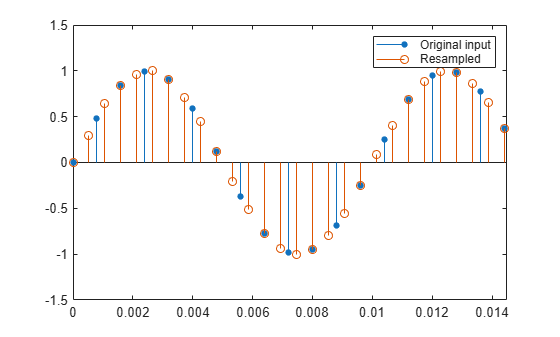Note: If you are using R2016a or an earlier release, replace each call to the object with the equivalent `step `syntax. For example, `obj(x) `becomes `step(obj,x)`.

Note: The `audioDeviceWriter` System object™ is not supported in MATLAB Online.

This example shows how to resample and play an audio signal from 48 kHz to 44 kHz on a Windows® platform.

Create a `dsp.FIRRateConverter` object with interpolation factor of L = 11 and decimation factor of M = 12 (the co-prime representation of the ratio $44\mathit{k}/48\mathit{k}$), a reasonable approximation of the standard 44.1 kHz rate. The FIR is a lowpass filter, designed using `designMultirateFIR` function for the specified conversion ratio.

```L = 11; M = 12; num = designMultirateFIR(L,M); firrc = dsp.FIRRateConverter(L,M,num);```

Initialize source and sink.

```afr = dsp.AudioFileReader('audio48kHz.wav', ... 'OutputDataType', 'single', ... 'SamplesPerFrame', 4*M); adw = audioDeviceWriter(44100);```

Read audio from the file, convert the rate of the signal, and play the converted audio.

```while ~isDone(afr) audio1 = afr(); audio2 = firrc(audio1); adw(audio2); end release(afr); release(adw);```

## Algorithms

The FIR rate converter is implemented efficiently using a polyphase structure.

To derive the polyphase structure, start with the transfer function of the FIR filter: This FIR filter is a combined anti-imaging and anti-aliasing filter.

`$H\left(z\right)={b}_{0}+{b}_{1}{z}^{-1}+...+{b}_{N}{z}^{-N}$`

N+1 is the length of the FIR filter.

You can rearrange this equation as follows:

`$H\left(z\right)=\begin{array}{c}\left({b}_{0}+{b}_{L}{z}^{-L}+{b}_{2L}{z}^{-2L}+..+{b}_{N-L+1}{z}^{-\left(N-L+1\right)}\right)+\\ {z}^{-1}\left({b}_{1}+{b}_{L+1}{z}^{-L}+{b}_{2L+1}{z}^{-2L}+..+{b}_{N-L+2}{z}^{-\left(N-L+1\right)}\right)+\\ \begin{array}{c}⋮\\ {z}^{-\left(L-1\right)}\left({b}_{L-1}+{b}_{2L-1}{z}^{-L}+{b}_{3L-1}{z}^{-2L}+..+{b}_{N}{z}^{-\left(N-L+1\right)}\right)\end{array}\end{array}$`

L is the number of polyphase components, and its value equals the interpolation factor that you specify.

You can write this equation as:

`$H\left(z\right)={E}_{0}\left({z}^{L}\right)+{z}^{-1}{E}_{1}\left({z}^{L}\right)+...+{z}^{-\left(L-1\right)}{E}_{L-1}\left({z}^{L}\right)$`

E0(zL), E1(zL), ..., EL-1(zL) are polyphase components of the FIR filter H(z).

Conceptually, the FIR rate converter contains an upsampler, followed by a combined anti-imaging, anti-aliasing FIR filter H(z), which is followed by a downsampler.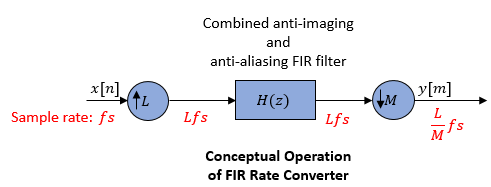Replace H(z) with its polyphase representation.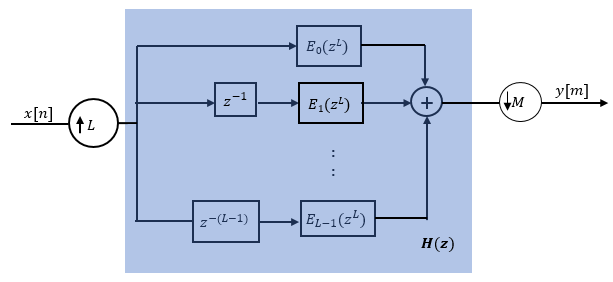Here is the multirate noble identity for interpolation.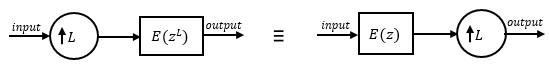Applying the noble identity for interpolation moves the upsampling operation to after the filtering operation. This move enables you to filter the signal at a lower rate.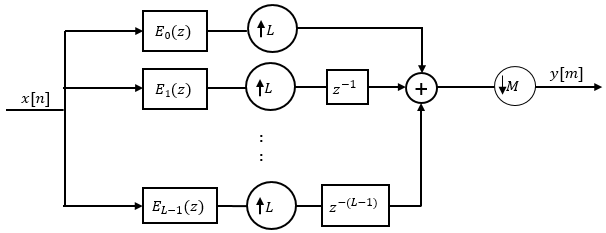You can replace the upsampling operator, delay block, and the adder with a commutator switch. To account for the downsampler that follows, the switch moves in steps of size M. The switch receives the first sample from branch 0 and moves in the counter clockwise direction, each time skipping M−1 branches.

As an example, consider a rate converter with L set to 5 and M set to 3. The polyphase components are E0(z), E1(z), E2(z), E3(z), and E4(z). The switch starts on the first branch 0, skips branches 1 and 2, receives the next sample from branch 3, then skips branches 4 and 0, receives the next sample from branch 2, and so on. The sequence of branches from which the switch receives the data sample is [0, 3, 1, 4, 2, 0, 3, 1, ….].

The rate converter implements the L/M conversion by first applying the interpolation factor L to the incoming data, and using the commutator switch at the end to receive only 1 in M samples, effectively accounting for the dowsampling factor M. Hence, the sample rate at the output of the FIR rate converter is Lfs/M.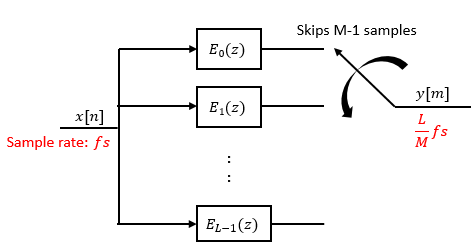## Extended Capabilities

### Fixed-Point ConversionDesign and simulate fixed-point systems using Fixed-Point Designer™.

Introduced in R2012a

Watch now